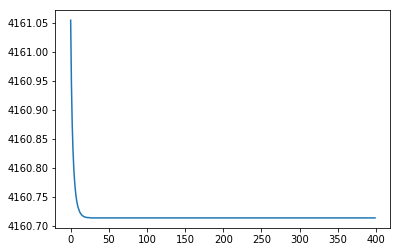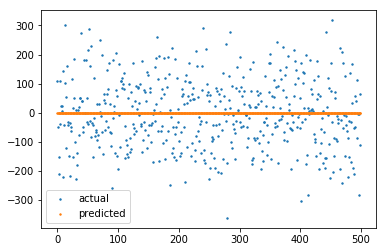# Why regression model built using torch wont work well?

I am quite new with torch.
And I have mostly difficulty with fitting simple feed forward network with some random data

``````class Net(torch.nn.Module):
def __init__(self, n_feature, n_hidden, n_output):
super(Net, self).__init__()
self.hidden = torch.nn.Linear(n_feature, n_hidden)
self.predict = torch.nn.Linear(n_hidden, n_output)

def forward(self, x):
x = F.relu(self.hidden(x))
x = self.predict(x)
return x
``````
``````import sklearn.datasets as datasets
X_,y_=datasets.make_regression(n_samples=500,n_features=2,noise=0.1)
X = torch.from_numpy(X_).float()
y = torch.from_numpy(y_).float()
net = Net(n_feature=2, n_hidden=10, n_output=1)
print(net)

optimizer = torch.optim.SGD(net.parameters(), lr=0.02)
loss_func = torch.nn.MSELoss()

for t in range(2000):
prediction = net(X)

loss = loss_func(prediction, y)

loss.backward()
optimizer.step()

if t % 5 == 0:
print(loss.data.numpy())
``````

I tried to tune hyper parameters like number of nodes and learning rate as well as tried changing optimiser and loss function but looks like not much improvement. Is there any issue in above code or someone also had bad time with torchs regression?

`optimizer.zero_grad()` should be calculated before `loss = loss_func(prediction, y)`

I tried rearranging zero grad before loss but but seems like loss not going much down though.In fact, `optimizer.zero_grad()` should be before `prediction = net(X)`

Also, try to reduce the learning rate, maybe using learning rate decay via scheduler

Yes that is what I tried so now

``````for t in range(2000):
prediction = net(X)

loss = loss_func(prediction, y)
loss.backward()
optimizer.step()

if t % 5 == 0:
print(loss.data.numpy())
``````

yes it is before prediction
and I just compared results from model with training data its still looks like not learning anythinghence loss is very high

Try this:

``````for t in range(2000):
prediction = net(X)
loss = loss_func(prediction, y)
loss.backward()
optimizer.step()

if t % 5 == 0:
print(loss.data.numpy())
``````

Also, you might need to lower the learning rate as I described above

``````losses=[]
scheduler = torch.optim.lr_scheduler.StepLR(optimizer, step_size=30, gamma=0.1)
for t in range(2000):
prediction = net(X)     # input x and predict based on x

loss = loss_func(prediction, y)

scheduler.step()      # change learning rate on every 30th iteration

if t % 5 == 0:
losses.append(loss.data.numpy())
``````

I already tried both ways but but still loss and prediction doesn’t change .
I think need some transformation over data.

You need thus to normalize your data to have zero mean and unit variance.

I am trying normalisation but I am still worried about why torch fails to learn anything? as most of prediction from model is around zero

Might be unrelated, but could you check if the model output and target have the same shape?
If your target is missing the feature dimension (`[batch_size]` instead of `[batch_size, 1]`), an unwanted broadcast might be applied.
@Deeply I did not mean the normalization, meant the part about the position of zero_grad